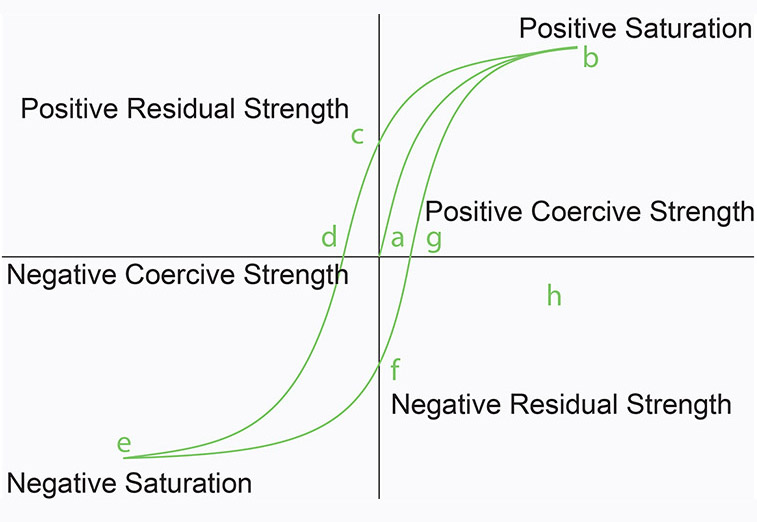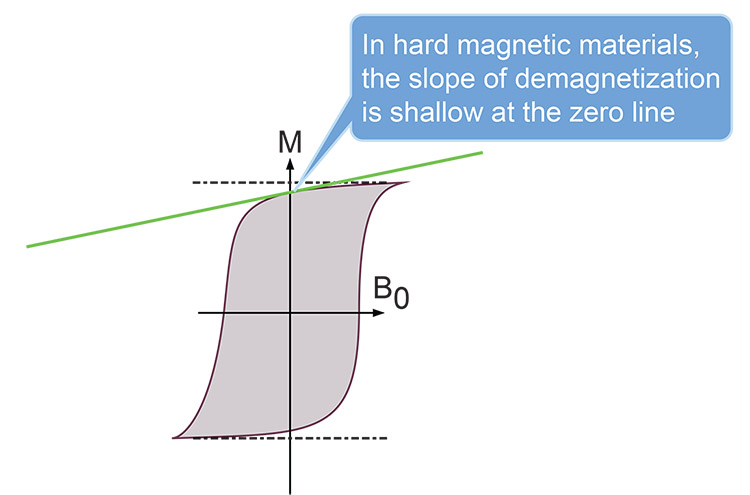# The Hysteresis Loop Explained- Understanding Hysteresis and BH Curves

## Hysteresis means lagging

Hysteresis is based on a Greek word that means lagging. So Magnetic Hysteresis is all about how a material's magnetic properties lag behind the force that creates those properties.  The hysteresis curve tells us a lot about a material's response to a magnetic field, so if we know how to interpret it, we will know and understand a lot about the material's response to magnetic input.

We plot a material's hysteresis on a graph known as the Hysteresis Loop.  Another way to express it is to say that a hysteresis loop shows the relationship between the external magnetizing force and the induced magnetic flux density.

## What is BH Curve?

The plot of Hysteresis is known as a B-H curve, where B (The Material's Flux Density, measured in Teslas or Mega Gauss) is plotted on the vertical axis and H (The External Applied Magnetizing Force, measured in Amperes per meter) is plotted on the horizontal axis.  We can also learn a number of other magnetic concepts and principles just by examining the hysteresis loop in detail.B-H Curve of Magnetic Material. As we follow the letters from a through g we generate important magnetic data about the material that helps us understand the material's magnetic properties.

The Flux Density (B) of a magnetic material will increase- from point a at the origin- in the presence of an applied magnetic field (H) until it reaches a maximum where it no longer responds to increases in the magnetic field at point b. This is the point of Positive Saturation, where any further increase in the external magnetic field will not increase the flux density of the material any further.

## Discovering Remanence and Coercivity in the Hysteresis Loop

The next thing we do is go back to the left along the horizontal axis toward the origin.  We find that the material releases some of its magnetism, and where the applied field has reached zero again, (point c on the B-H Curve) the material finds its residual magnetic strength, which is called Remanence (also known as Retentivity).

### What is Remanence?

Remanence is the remaining magnetic field found in the material after the applied magnetic field is reduced to zero.  Based on the raw value of Remanence and the shape of the curve we are generating with the applied magnetic field, we can determine whether the specimen under study is a hard- or soft magnetic material.

As we now reverse the applied magnetic field (H)and drive it to the left, we see the value of B (Flux Density of the material) decline to zero.  We are now at point d on the B-H Curve.

### What is Coercivity?

The amount of (H) required to drive (B) to the zero line gives us a value for the material's Coercivity. Coercivity -also known as Coercive Strength- is the resistance of a material to changes in magnetization.  Soft magnetic materials tend to have low Coercivity and hard magnetic materials have high Coercivity. Regardless of the magnetic hardness or softness of the material, point d defines the moment that they have lost their magnetic strength.

## The Shape of the B-H Curve in Hard- and Soft Magnetic Materials

Soft magnetic materials have a thin hysteresis curve, so they see a lot of usage in applications that require them to switch polarity often, such as in transformers and motor windings. As seen below, soft magnetic materials have low Remanence, and have already lost a large percentage of their magnetic field by the time they cross the zero line. They also have a steep negative slope of demagnetization at the zero line.

Soft magnetic materials -with their low Coercivity- can switch polarity often and sustain relatively small electrical losses.  The width of the hysteresis loop tells us a lot about the losses.  The narrower the curve, the lower the losses are.Hard magnetic materials have a very wide hysteresis curve, which makes them practical in applications where they exert their magnetic field on soft magnetic materials. As seen in the figure below, hard magnetic materials have high Remanence, and have lost only a small percentage of their magnetic field by the time they cross the zero line. Their slope of demagnetization at the zero line is very shallow and does not steepen until it goes far to the left of the zero line.  If hard magnetic materials were to change polarity often , the hysteresis losses would be huge, so they are not used in that manner.## Negative Saturation

Pushing to the left on the B axis, we continue to increase the magnetic field in the opposite direction until reaching point e -the point of Negative Saturation.  This point is just like point b -but in the opposite direction.  Whether hard or soft, the magnetic material in our study is now magnetized in the opposite direction of its magnetization at point b.

If we continue back to point b from point e, we will simply do the same thing over again that we originally did -but in the opposite direction.

Referring to Figure 1, where we began, we see how we have traveled all the way around the hysteresis loop.  But there's one thing left to note.  Due to Remanence, we will never get back to point a where we started, because point a was the point where the material had never been exposed to a magnetic field.

Would you like to study this material in more depth? A very good video tutorial is available here